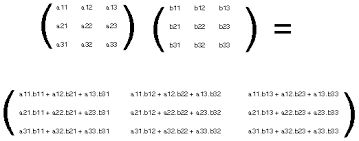Q

# Solve it, - Matrices and Determinants - JEE Main-3

If A and B are square matrices of size n x n such that then which of the following will be always true?

• Option 1)

A = B

• Option 2)

AB = BA

• Option 3)

either A or B is a zero matrix

• Option 4)

either A or B is an identity matrix

120 Views
N

As we learn tin

Multiplication of matrices --

Option 1)

A = B

Incorrect option

Option 2)

AB = BA

Correct option

Option 3)

either A or B is a zero matrix

Incorrect option

Option 4)

either A or B is an identity matrix

Incorrect option

Exams
Articles
Questions